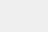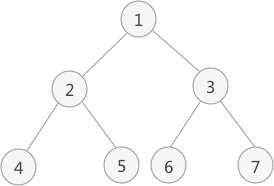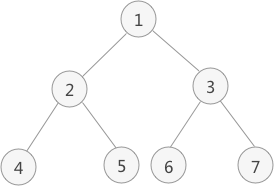• 二叉树中序遍历的实现思想是：访问当前节点的左子树；访问根节点；访问当前节点的右子树；图 1 二叉树以图 1 为例，采用中序遍历的思想遍历该二叉树的过程为：访问该二叉树的根节点，找到 1；遍历节点 1 的左子树，...

二叉树中序遍历的实现思想是：访问当前节点的左子树；

访问根节点；

访问当前节点的右子树；图 1 二叉树

以图  1 为例，采用中序遍历的思想遍历该二叉树的过程为：访问该二叉树的根节点，找到 1；

遍历节点 1 的左子树，找到节点 2；

遍历节点 2 的左子树，找到节点 4；

由于节点 4 无左孩子，因此找到节点 4，并遍历节点 4 的右子树；

由于节点 4 无右子树，因此节点 2 的左子树遍历完成，访问节点 2；

遍历节点 2 的右子树，找到节点 5；

由于节点 5 无左子树，因此访问节点 5 ，又因为节点 5 没有右子树，因此节点 1 的左子树遍历完成，访问节点 1 ，并遍历节点 1 的右子树，找到节点 3；

遍历节点 3 的左子树，找到节点 6；

由于节点 6 无左子树，因此访问节点 6，又因为该节点无右子树，因此节点 3 的左子树遍历完成，开始访问节点 3 ，并遍历节点 3 的右子树，找到节点 7；

由于节点 7 无左子树，因此访问节点 7，又因为该节点无右子树，因此节点 1 的右子树遍历完成，即整棵树遍历完成；

因此，图 1 中二叉树采用中序遍历得到的序列为：

4 2 5 1 6 3 7

递归实现

二叉树的中序遍历采用的是递归的思想，因此可以递归实现，其 C 语言实现代码为：#include #include #define TElemType int //构造结点的结构体 typedef struct BiTNode{ TElemType data;//数据域 struct BiTNode *lchild,*rchild;//左右孩子指针 }BiTNode,*BiTree; //初始化树的函数 void CreateBiTree(BiTree *T){ *T=(BiTNode*)malloc(sizeof(BiTNode)); (*T)->data=1; (*T)->lchild=(BiTNode*)malloc(sizeof(BiTNode)); (*T)->rchild=(BiTNode*)malloc(sizeof(BiTNode)); (*T)->lchild->data=2; (*T)->lchild->lchild=(BiTNode*)malloc(sizeof(BiTNode)); (*T)->lchild->rchild=(BiTNode*)malloc(sizeof(BiTNode)); (*T)->lchild->rchild->data=5; (*T)->lchild->rchild->lchild=NULL; (*T)->lchild->rchild->rchild=NULL; (*T)->rchild->data=3; (*T)->rchild->lchild=(BiTNode*)malloc(sizeof(BiTNode)); (*T)->rchild->lchild->data=6; (*T)->rchild->lchild->lchild=NULL; (*T)->rchild->lchild->rchild=NULL; (*T)->rchild->rchild=(BiTNode*)malloc(sizeof(BiTNode)); (*T)->rchild->rchild->data=7; (*T)->rchild->rchild->lchild=NULL; (*T)->rchild->rchild->rchild=NULL; (*T)->lchild->lchild->data=4; (*T)->lchild->lchild->lchild=NULL; (*T)->lchild->lchild->rchild=NULL; } //模拟操作结点元素的函数，输出结点本身的数值 void displayElem(BiTNode* elem){ printf("%d ",elem->data); } //中序遍历 void INOrderTraverse(BiTree T){ if (T) { INOrderTraverse(T->lchild);//遍历左孩子 displayElem(T);//调用操作结点数据的函数方法 INOrderTraverse(T->rchild);//遍历右孩子 } //如果结点为空，返回上一层 return; } int main() { BiTree Tree; CreateBiTree(&Tree); printf("中序遍历算法: n"); INOrderTraverse(Tree); }

运行结果：

中序遍历算法:

4 2 5 1 6 3 7

非递归实现

而递归的底层实现依靠的是栈存储结构，因此，二叉树的先序遍历既可以直接采用递归思想实现，也可以使用栈的存储结构模拟递归的思想实现。

中序遍历的非递归方式实现思想是：从根结点开始，遍历左孩子同时压栈，当遍历结束，说明当前遍历的结点没有左孩子，从栈中取出来调用操作函数，然后访问该结点的右孩子，继续以上重复性的操作。

除此之外，还有另一种实现思想：中序遍历过程中，只需将每个结点的左子树压栈即可，右子树不需要压栈。当结点的左子树遍历完成后，只需要以栈顶结点的右孩子为根结点，继续循环遍历即可。

两种非递归方法实现二叉树中序遍历的代码实现为：#include #include #define TElemType int int top=-1;//top变量时刻表示栈顶元素所在位置 //构造结点的结构体 typedef struct BiTNode{ TElemType data;//数据域 struct BiTNode *lchild,*rchild;//左右孩子指针 }BiTNode,*BiTree; //初始化树的函数 void CreateBiTree(BiTree *T){ *T=(BiTNode*)malloc(sizeof(BiTNode)); (*T)->data=1; (*T)->lchild=(BiTNode*)malloc(sizeof(BiTNode)); (*T)->rchild=(BiTNode*)malloc(sizeof(BiTNode)); (*T)->lchild->data=2; (*T)->lchild->lchild=(BiTNode*)malloc(sizeof(BiTNode)); (*T)->lchild->rchild=(BiTNode*)malloc(sizeof(BiTNode)); (*T)->lchild->rchild->data=5; (*T)->lchild->rchild->lchild=NULL; (*T)->lchild->rchild->rchild=NULL; (*T)->rchild->data=3; (*T)->rchild->lchild=(BiTNode*)malloc(sizeof(BiTNode)); (*T)->rchild->lchild->data=6; (*T)->rchild->lchild->lchild=NULL; (*T)->rchild->lchild->rchild=NULL; (*T)->rchild->rchild=(BiTNode*)malloc(sizeof(BiTNode)); (*T)->rchild->rchild->data=7; (*T)->rchild->rchild->lchild=NULL; (*T)->rchild->rchild->rchild=NULL; (*T)->lchild->lchild->data=4; (*T)->lchild->lchild->lchild=NULL; (*T)->lchild->lchild->rchild=NULL; } //前序和中序遍历使用的进栈函数 void push(BiTNode** a,BiTNode* elem){ a[++top]=elem; } //弹栈函数 void pop( ){ if (top==-1) { return ; } top--; } //模拟操作结点元素的函数，输出结点本身的数值 void displayElem(BiTNode* elem){ printf("%d ",elem->data); } //拿到栈顶元素 BiTNode* getTop(BiTNode**a){ return a[top]; } //中序遍历非递归算法 void InOrderTraverse1(BiTree Tree){ BiTNode* a;//定义一个顺序栈 BiTNode * p;//临时指针 push(a, Tree);//根结点进栈 while (top!=-1) {//top!=-1说明栈内不为空，程序继续运行 while ((p=getTop(a)) &&p){//取栈顶元素，且不能为NULL push(a, p->lchild);//将该结点的左孩子进栈，如果没有左孩子，NULL进栈 } pop();//跳出循环，栈顶元素肯定为NULL，将NULL弹栈 if (top!=-1) { p=getTop(a);//取栈顶元素 pop();//栈顶元素弹栈 displayElem(p); push(a, p->rchild);//将p指向的结点的右孩子进栈 } } } //中序遍历实现的另一种方法 void InOrderTraverse2(BiTree Tree){ BiTNode* a;//定义一个顺序栈 BiTNode * p;//临时指针 p=Tree; //当p为NULL或者栈为空时，表明树遍历完成 while (p || top!=-1) { //如果p不为NULL，将其压栈并遍历其左子树 if (p) { push(a, p); p=p->lchild; } //如果p==NULL，表明左子树遍历完成，需要遍历上一层结点的右子树 else{ p=getTop(a); pop(); displayElem(p); p=p->rchild; } } } int main(){ BiTree Tree; CreateBiTree(&Tree); printf("中序遍历算法1: n"); InOrderTraverse1(Tree); printf("n中序遍历算法2: n"); InOrderTraverse2(Tree); }

运行结果

中序遍历算法1:

4 2 5 1 6 3 7

中序遍历算法2:

4 2 5 1 6 3 7原文始发于：二叉树中序遍历(递归和非递归)算法C语言实现

展开全文• 该楼层疑似违规已被系统折叠隐藏此楼查看此楼我以前的代码有实现前序和后序的，中序感觉应该差不多struct AvlTreeNode* AvlTreeIterateHead(struct AvlTree* tree){struct AvlTreeNode* currentNode = tree->...

该楼层疑似违规已被系统折叠 隐藏此楼查看此楼

我以前的代码有实现前序和后序的，中序感觉应该差不多

{

struct AvlTreeNode* currentNode = tree->m_rootNode;

if(currentNode != tree->m_nullNode)

{

// find the smallest node

while(currentNode->m_child1 != tree->m_nullNode)

currentNode = currentNode->m_child1;

return currentNode;

}

return NULL;

}

struct AvlTreeNode* AvlTreeIterateTail(struct AvlTree* tree)

{

struct AvlTreeNode* currentNode = tree->m_rootNode;

if(currentNode != tree->m_nullNode)

{

// find the greatest node

while(currentNode->m_child2 != tree->m_nullNode)

currentNode = currentNode->m_child2;

return currentNode;

}

return NULL;

}

struct AvlTreeNode* AvlTreeIterateNext(

struct AvlTree* tree,

struct AvlTreeNode* node)

{

struct AvlTreeNode* currentNode;

if(node->m_child2 != tree->m_nullNode)

{

// look for right child's smallest node

currentNode = node->m_child2;

while(currentNode->m_child1 != tree->m_nullNode)

currentNode = currentNode->m_child1;

return currentNode;

}

else if(node->m_parent != tree->m_nullNode)

{

currentNode = node;

// the node has no right child, look back

while(currentNode != tree->m_rootNode)

if(currentNode->m_parent->m_child2 != currentNode)

return currentNode->m_parent;

else currentNode = currentNode->m_parent;

}

return NULL;

}

struct AvlTreeNode* AvlTreeIteratePrev(

struct AvlTree* tree,

struct AvlTreeNode* node)

{

struct AvlTreeNode* currentNode;

if(node->m_child1 != tree->m_nullNode)

{

currentNode = node->m_child1;

// look for left child's greatest node

while(currentNode->m_child2 != tree->m_nullNode)

currentNode = currentNode->m_child2;

return currentNode;

}

else if(node->m_parent != tree->m_nullNode)

{

currentNode = node;

// the node has no left child, look back

while(currentNode != tree->m_rootNode)

if(currentNode->m_parent->m_child1 != currentNode)

return currentNode->m_parent;

else currentNode = currentNode->m_parent;

}

return NULL;

}

展开全文• 二叉树中序遍历的实现思想是： 访问当前节点的左子树； 访问根节点； 访问当前节点的右子树； 图 1 二叉树 以图 1 为例，采用中序遍历的思想遍历该二叉树的过程为： 访问该二叉树的根节点，找到 1； 遍历节点 1...

二叉树中序遍历的实现思想是：

1. 访问当前节点的左子树；
2. 访问根节点；
3. 访问当前节点的右子树；图 1 二叉树以图  1 为例，采用中序遍历的思想遍历该二叉树的过程为：

1. 访问该二叉树的根节点，找到 1；
2. 遍历节点 1 的左子树，找到节点 2；
3. 遍历节点 2 的左子树，找到节点 4；
4. 由于节点 4 无左孩子，因此找到节点 4，并遍历节点 4 的右子树；
5. 由于节点 4 无右子树，因此节点 2 的左子树遍历完成，访问节点 2；
6. 遍历节点 2 的右子树，找到节点 5；
7. 由于节点 5 无左子树，因此访问节点 5 ，又因为节点 5 没有右子树，因此节点 1 的左子树遍历完成，访问节点 1 ，并遍历节点 1 的右子树，找到节点 3；
8. 遍历节点 3 的左子树，找到节点 6；
9. 由于节点 6 无左子树，因此访问节点 6，又因为该节点无右子树，因此节点 3 的左子树遍历完成，开始访问节点 3 ，并遍历节点 3 的右子树，找到节点 7；
10. 由于节点 7 无左子树，因此访问节点 7，又因为该节点无右子树，因此节点 1 的右子树遍历完成，即整棵树遍历完成；

因此，图 1 中二叉树采用中序遍历得到的序列为：

4 2 5 1 6 3 7

递归实现

二叉树的中序遍历采用的是递归的思想，因此可以递归实现，其 C 语言实现代码为：

#include <stdio.h>
#include <string.h>
#define TElemType int
//构造结点的结构体
typedef struct BiTNode{
TElemType data;//数据域
struct BiTNode *lchild,*rchild;//左右孩子指针
}BiTNode,*BiTree;
//初始化树的函数
void CreateBiTree(BiTree *T){
*T=(BiTNode*)malloc(sizeof(BiTNode));
(*T)->data=1;
(*T)->lchild=(BiTNode*)malloc(sizeof(BiTNode));
(*T)->rchild=(BiTNode*)malloc(sizeof(BiTNode));

(*T)->lchild->data=2;
(*T)->lchild->lchild=(BiTNode*)malloc(sizeof(BiTNode));
(*T)->lchild->rchild=(BiTNode*)malloc(sizeof(BiTNode));
(*T)->lchild->rchild->data=5;
(*T)->lchild->rchild->lchild=NULL;
(*T)->lchild->rchild->rchild=NULL;
(*T)->rchild->data=3;
(*T)->rchild->lchild=(BiTNode*)malloc(sizeof(BiTNode));
(*T)->rchild->lchild->data=6;
(*T)->rchild->lchild->lchild=NULL;
(*T)->rchild->lchild->rchild=NULL;
(*T)->rchild->rchild=(BiTNode*)malloc(sizeof(BiTNode));
(*T)->rchild->rchild->data=7;
(*T)->rchild->rchild->lchild=NULL;
(*T)->rchild->rchild->rchild=NULL;
(*T)->lchild->lchild->data=4;
(*T)->lchild->lchild->lchild=NULL;
(*T)->lchild->lchild->rchild=NULL;
}
//模拟操作结点元素的函数，输出结点本身的数值
void displayElem(BiTNode* elem){
printf("%d ",elem->data);
}
//中序遍历
void INOrderTraverse(BiTree T){
if (T) {
INOrderTraverse(T->lchild);//遍历左孩子
displayElem(T);//调用操作结点数据的函数方法
INOrderTraverse(T->rchild);//遍历右孩子
}
//如果结点为空，返回上一层
return;
}
int main() {
BiTree Tree;
CreateBiTree(&Tree);
printf("中序遍历算法: \n");
INOrderTraverse(Tree);
}

运行结果：
中序遍历算法:
4 2 5 1 6 3 7

非递归实现

而递归的底层实现依靠的是栈存储结构，因此，二叉树的先序遍历既可以直接采用递归思想实现，也可以使用栈的存储结构模拟递归的思想实现。
中序遍历的非递归方式实现思想是：从根结点开始，遍历左孩子同时压栈，当遍历结束，说明当前遍历的结点没有左孩子，从栈中取出来调用操作函数，然后访问该结点的右孩子，继续以上重复性的操作。
除此之外，还有另一种实现思想：中序遍历过程中，只需将每个结点的左子树压栈即可，右子树不需要压栈。当结点的左子树遍历完成后，只需要以栈顶结点的右孩子为根结点，继续循环遍历即可。
两种非递归方法实现二叉树中序遍历的代码实现为：

#include <stdio.h>
#include <string.h>
#define TElemType int
int top=-1;//top变量时刻表示栈顶元素所在位置
//构造结点的结构体
typedef struct BiTNode{
TElemType data;//数据域
struct BiTNode *lchild,*rchild;//左右孩子指针
}BiTNode,*BiTree;
//初始化树的函数
void CreateBiTree(BiTree *T){
*T=(BiTNode*)malloc(sizeof(BiTNode));
(*T)->data=1;
(*T)->lchild=(BiTNode*)malloc(sizeof(BiTNode));
(*T)->rchild=(BiTNode*)malloc(sizeof(BiTNode));
(*T)->lchild->data=2;
(*T)->lchild->lchild=(BiTNode*)malloc(sizeof(BiTNode));
(*T)->lchild->rchild=(BiTNode*)malloc(sizeof(BiTNode));
(*T)->lchild->rchild->data=5;
(*T)->lchild->rchild->lchild=NULL;
(*T)->lchild->rchild->rchild=NULL;
(*T)->rchild->data=3;
(*T)->rchild->lchild=(BiTNode*)malloc(sizeof(BiTNode));
(*T)->rchild->lchild->data=6;
(*T)->rchild->lchild->lchild=NULL;
(*T)->rchild->lchild->rchild=NULL;
(*T)->rchild->rchild=(BiTNode*)malloc(sizeof(BiTNode));
(*T)->rchild->rchild->data=7;
(*T)->rchild->rchild->lchild=NULL;
(*T)->rchild->rchild->rchild=NULL;
(*T)->lchild->lchild->data=4;
(*T)->lchild->lchild->lchild=NULL;
(*T)->lchild->lchild->rchild=NULL;
}
//前序和中序遍历使用的进栈函数
void push(BiTNode** a,BiTNode* elem){
a[++top]=elem;
}
//弹栈函数
void pop( ){
if (top==-1) {
return ;
}
top--;
}
//模拟操作结点元素的函数，输出结点本身的数值
void displayElem(BiTNode* elem){
printf("%d ",elem->data);
}
//拿到栈顶元素
BiTNode* getTop(BiTNode**a){
return a[top];
}
//中序遍历非递归算法
void InOrderTraverse1(BiTree Tree){
BiTNode* a;//定义一个顺序栈
BiTNode * p;//临时指针
push(a, Tree);//根结点进栈
while (top!=-1) {//top!=-1说明栈内不为空，程序继续运行
while ((p=getTop(a)) &&p){//取栈顶元素，且不能为NULL
push(a, p->lchild);//将该结点的左孩子进栈，如果没有左孩子，NULL进栈
}
pop();//跳出循环，栈顶元素肯定为NULL，将NULL弹栈
if (top!=-1) {
p=getTop(a);//取栈顶元素
pop();//栈顶元素弹栈
displayElem(p);
push(a, p->rchild);//将p指向的结点的右孩子进栈
}
}
}
//中序遍历实现的另一种方法
void InOrderTraverse2(BiTree Tree){
BiTNode* a;//定义一个顺序栈
BiTNode * p;//临时指针
p=Tree;
//当p为NULL或者栈为空时，表明树遍历完成
while (p || top!=-1) {
//如果p不为NULL，将其压栈并遍历其左子树
if (p) {
push(a, p);
p=p->lchild;
}
//如果p==NULL，表明左子树遍历完成，需要遍历上一层结点的右子树
else{
p=getTop(a);
pop();
displayElem(p);
p=p->rchild;
}
}
}
int main(){
BiTree Tree;
CreateBiTree(&Tree);
printf("中序遍历算法1: \n");
InOrderTraverse1(Tree);
printf("\n中序遍历算法2: \n");
InOrderTraverse2(Tree);
}

运行结果

中序遍历算法1:
4 2 5 1 6 3 7
中序遍历算法2:
4 2 5 1 6 3 7

展开全文二叉树 指针 数据结构 算法 java
• 利用栈的基本操作实现二叉树的中序遍历递归算法数据结构
• 94. 二叉树的中序遍历 – 非递归实现 // code ver1 还是很直观易懂的 int* inorderTraversal(struct TreeNode* root, int* returnSize){ int* ans; ans = (int *)malloc(sizeof(int) * MAXSIZE); int i = 0; if...

94. 二叉树的中序遍历 – 非递归实现

// code ver1 还是很直观易懂的
int* inorderTraversal(struct TreeNode* root, int* returnSize){
int* ans;
ans = (int *)malloc(sizeof(int) * MAXSIZE);
int i = 0;

if(!root){
*returnSize = 0;
return ans;
}

SqStack* S = CreateStack();
//如果节点有左孩子，则入栈
while(root){
Push(S, root);
root = root->left;
}

//每弹出一个节点的同时，检查其是否有右孩子，有的话就存入该节点的右孩子以及该孩子的左孩子...
while(!StackEmpty(S)){
struct TreeNode* temp = StackPeek(S);
ans[i++] = Pop(S);

if(temp->right){
temp = temp->right;
Push(S, temp);

while(temp->left){
Push(S, temp->left);
temp = temp->left;
}
}
}

*returnSize = i;
return ans;
}

作者：zywang714
链接：https://leetcode-cn.com/problems/binary-tree-inorder-traversal/solution/zhi-chi-cyu-yan-by-jzns7jsmj6-2/
来源：力扣（LeetCode）
著作权归作者所有。商业转载请联系作者获得授权，非商业转载请注明出处。

// code ver2  优化后的cpp
class Solution {
public:
vector<int> inorderTraversal(TreeNode* root) {
stack<TreeNode*> s;
vector<int> res;
TreeNode* pnode=root;
while(!s.empty()||pnode!=NULL){
while(pnode!=NULL){
s.push(pnode);
pnode=pnode->left;
}
pnode=s.top();
res.push_back(pnode->val);
s.pop();
pnode=pnode->right;
}
return res;
}
};

作者：leo-503
链接：https://leetcode-cn.com/problems/binary-tree-inorder-traversal/solution/cc-shuang-100-zhan-die-dai-by-leo-503/
来源：力扣（LeetCode）
著作权归作者所有。商业转载请联系作者获得授权，非商业转载请注明出处。

#define MAXSIZE 1000

int* inorderTraversal(struct TreeNode* root, int* returnSize){

struct TreeNode *p[MAXSIZE];
int top = -1,i= 0;
int *r;
r = (int *)malloc(sizeof(int)*MAXSIZE);

struct TreeNode *temp ;
temp = root;

while(temp != NULL || top != -1)
{
if(temp != NULL)
{
top++;
p[top] = temp;
temp = temp->left;
}
else
{
temp = p[top];
top--;
r[i] = temp->val;
i++;
temp = temp->right;
}
}
(*returnSize) = i;
return r;
}

作者：andy2333-4
链接：https://leetcode-cn.com/problems/binary-tree-inorder-traversal/solution/jing-dian-zhan-jie-jue-er-cha-shu-qian-zhong-hou-x/
来源：力扣（LeetCode）
著作权归作者所有。商业转载请联系作者获得授权，非商业转载请注明出处。

// code ver3
int* inorderTraversal(struct TreeNode* root, int* returnSize){

int size=TreeSize(root);
//memset(res,0,size);

int p=-1,index=0;
if(!root){
*returnSize=0;
return NULL;
}
struct TreeNode** src=malloc(sizeof(struct TreeNode*)*size);
int *res=malloc(sizeof(int)*size);
while(root!=NULL||p!=-1)
{
while(root)
{
src[++p]=root;
root=root->left;//一直往左走
}

if(p!=-1) {
root=src[p--];
res[index++]=root->val;
root=root->right;
}
}
*returnSize =index;
return res;
}

作者：cocowy
链接：https://leetcode-cn.com/problems/binary-tree-inorder-traversal/solution/da-dao-zhi-jian-dong-tai-shen-qing-shu-zu-di-gui-q/
来源：力扣（LeetCode）
著作权归作者所有。商业转载请联系作者获得授权，非商业转载请注明出处。

int* inorderTraversal(struct TreeNode* root, int* returnSize){
int *arr = NULL;
struct TreeNode *stack = {}, *node;
int top = 0;
*returnSize = 0;
if (!root)  return arr;
arr = malloc(sizeof(int) * 50000);
node = root;
while (top || node) {
while (node) {
stack[top++] = node;
// printf("%d\n",node->val);
node = node->left;
}
if (top) {
node = stack[--top];
arr[(*returnSize)++] = node->val;
// if (node->right)
node = node->right;
// else
// node = NULL;
}
}
return arr;
}

展开全文• 自己编写的实验二叉树的后序遍历递归算法 包括以递归中序遍历建立二叉树 前序，中序，后序递归以及非递归实现二叉树的遍历 经vc6.0编译通过 自己实验，不足之处应该很多，望指出
• 链式存储结构存储的递归思想遍历二叉树之前讲过，树是由根结点和子树部分...遍历左子树，之后访问根结点，然后遍历右子树，称为“中序遍历”；遍历完左右子树，再访问根结点，称为“后序遍历”。三种方式唯一的不同...
• // 最后先序遍历右子树 } } void InOrderTraverse(BiTree T,void(*Visit)(int)) { // 初始条件：二叉树T存在，Visit是对结点操作的应用函数 // 操作结果：中序递归遍历T，对每个结点调用函数Visit一次且仅一次 if(T...
• 递归先序算法建立二叉树。要求通过键盘输入二叉树的先序遍历顺序从而建立一棵二叉树。利用栈实现一棵二叉树的中序递归遍历。要求显示遍历次序。
• 中序遍历二叉排序树 输入一整数序列，建立二叉排序树，然后中序遍历。 输入说明 输入第一行为整数的个数n，第二行是具体的n个整数。 输出说明 建立二叉排序树，然后输出中序遍历的结果。 输入样例 5 1 6 5 9 8 ...数据结构
• 小小学习，C语言数据结构，中序遍历二叉树非递归算法中序 遍历
• //递归创建右子树 } } void InOrderTraverse(BiTree T)//中序遍历二叉树T的非递归算法 { LinkStack S; InitStack(S);//创建一个栈，并初始化 BiTree p = T; BiTree q = (BiTNode*)malloc(sizeof(BiTNode));//...二叉树 链表 数据结构 非递归 中序遍历
• C语言实现二叉树的中序遍历（非递归），本人亲自写的！
• // // Inordertraverse.c // 二叉树链式存储 // // Created by 丘** on 2021/7/28. // #include "Inordertraverse.h" #include <stdbool.h> #include<stdlib.h> typedef struct ... ...
• 用C++写的，包括二叉树的构建，二叉树的先序遍历中序遍历和后序遍历递归算法
• 数据结构双语 项目文档报告 用两种方式实现表达式自动计算 专 业 ....12 文档 实用标准文案 一设计思想 二叉树的遍历分为三种方式 分别是先序遍历 中序遍历和后序遍历 先序遍历实现的 顺序是根左右中序遍历实现的是左根
• 二叉排序树的定义 二叉排序树，也称二叉查找树。二叉排序树或者是一棵空树，或者是一颗具有以下特性的非空二叉树： ...右子树结点值，对二叉树中序遍历可得到一个递增的有序序列。 二叉排序树的创建...二叉排序树 数据结构 中序遍历
• 编写程序，用先序递归的方法建立二叉树，建立二叉树后，用中序递归方法遍历该二叉树，并输出遍历序列。二叉树
• 中序遍历二叉树（非递归算法c语言）

千次阅读 多人点赞 2015-07-24 19:28:56
void inOrderNo(BiTNode * T)//非递归进行中序遍历,借助于栈 {  BiTNode * temp=T;  Stack *s;  InitStack(s);  while(temp!=NULL||!isEmptyStack(s))  {  if(temp!=NULL)  {  if(push(s,...二叉树 遍历 非递归算法 队列
• 二叉树中序遍历(非递归)算法实现--C语言

万次阅读 多人点赞 2018-12-14 10:50:25
中序遍历的非递归算法有两种，但是个人觉得只要掌握一种就可以了，只要自己的逻辑清晰，会哪一种又有什么关系呢~ 首先给出今天的二叉树的示例图： 代码如下： // InOrderBiTreeTraverse.cpp : Defines the entry ...二叉树 中序遍历 非递归 C语言 数据结构
• 递归方法实现二叉树的中序遍历和后序遍历算法 //用递归方法实现二叉树的中序遍历和后序遍历算法； #include "stdio.h" #include "stdlib.h" #define OK 1 #define ERROR 0 #define OVERFLOW -2 typedef char ...算法 c语言 数据结构 二叉树
• #include #include ...//前序遍历：ABD#E##FG###C## typedef struct tree/*二叉树*/ { char data;  struct tree *lchild,*rchild; }bintree; typedef bintree *tree; tree createTree()/*前序遍历建树*C语言版数据结构...

中序遍历递归算法c语言c语言 订阅